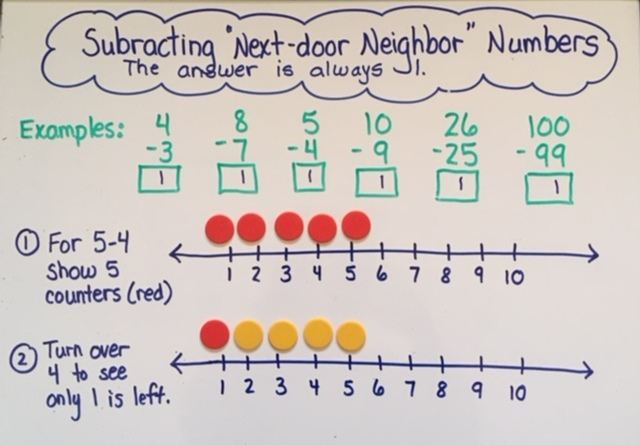# Addition and Subtraction Part 3: Facts Strategies KG-3rd

by C. Elkins, OK Math and Reading LadyThis is part three in a series of strategies regarding addition and subtraction strategies.  This part will focus on a variety of strategies to help toward memorization of facts, meaning automatic computation. While children are learning their number bonds (building up to 5 in KG, to 10 in first grade, and to 20 in second grade), there are other facts which cross several number bonds that students can work towards. These strategies to build mental math automaticity are highlighted below. Get some freebies in the section on doubles / near doubles.

Identity (or Zero) Property:

• The value of the number does not change when zero is added or subtracted.
• 3 + 0 = 3
• 9 – 0 = 9

Subtracting All:

• The answer is always zero when you take away / subtract all.
• 9 – 9 = 0
• 50 – 50 = 0

Adding 1 or Subtracting 1:

• Adding 1 results in the next number in the counting sequence.
• Subtracting 1 means naming the number that comes right before it in the counting sequence.
• With manipulatives, lay out an amount for student to count.  Slide one more and see if he/she can name the amount without recounting.
• Do the same as above, but take one away from the group to see if he/she can name the amount without recounting.
• Show this concept using a number line.
• 6 + 1 = 7;    26 + 1 = 27
• 7 – 1 = 6;     37 – 1 = 36
• After +1 or -1 strategies are in place, then go for +2 or -2 for automatic processing.

Next-Door Neighbor Numbers:

• If subtracting two sequential numbers (ie 7 subtract 6), the answer is always one because you are taking away almost all of the original amount.
• Help students identify these types of problems:  8-7;   10-9;   98-97;  158-157
• Guide students to writing these types of problems.
• Relate these to subtracting 1 problems.  If 10-1 = 9;   then 10 – 9 = 1.
• Show on a number line.Doubles (with freebies):

Near Doubles:

• After learning the doubles facts, work on doubles + 1 or doubles – 1 facts.
• If 3 + 3 = 6;  then 3 + 4 = 7   (six plus one more)
• If 4 + 4 = 8;  then 3 + 4 = 7  (eight minus one)
• I can picture this with a ten frame.
• See some freebies in above section.

Adding 10, 20, 30 . . . to a one digit number

• Start with problems such as 10 + 1, 10 + 2, 10 + 3, etc.  This shows a basic understanding of the teen numbers and place value.  Use the “cover zero” method. For example, to add 10 + 3, hold up a 10 card in your right hand (facing students) and a 3 card in your left hand.  Slide the 3 card over the zero of the 10 card so it looks like 13.
• Then move to other multiples of 10 such as 20 + 5 = 25, 30 + 8 = 38, 40 + 1 = 41, etc.
• Make sure students can also decompose these types of numbers.  14 = 10 and 4;  38 = 30 and 8
• Wait until their place value concepts are stronger to work on adding 10 to any 2 or 3 digit number or to subtract 10 from any 2 digit number.

Note:  Most of us (me included) tend to write math problems in this format a + b = ____ which is the “result unknown” format. When we write it as a + ___ = c  or __ + b = c you will most likely notice that students will add the two shown numbers together (because they see the plus sign) and put the sum in the blank, no matter where the blank appears. (So for this problem:  5 + ___ = 8, the child is likely to put 13 in the blank.) This is because they do not see the function of the equal sign.  The equal sign does not mean “the sum goes here.” The equal sign means “the same as.”  What is on the left of the equal sign must equal what is on the right of the equal sign.  To help with this, alter your equations to reflect different positions of the missing addend. In next week’s post, I will address some strategies to deal with these types of problems (change unknown, start unknown) and how they relate to counting up and subtraction . . . so stay tuned!!

Would love to hear from some of you! Comments always welcomed . . . share your thoughts or your strategies.